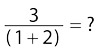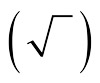##### 1,001 ASVAB Practice Questions For Dummies (+ Free Online Practice)One of the most basic things you should know for the Mathematics Knowledge subtest of the ASVAB is the order of operations. Operations must be performed in a certain order. For example, when you have parentheses in a math problem, the calculation in the parentheses must be done before any calculations outside of the parentheses.

In the equation 2 × (16 + 5) = ?, you first add 16 to 5 to arrive at 21, and then you multiply by 2 to come up with a total of 42. You get a different (and wrong) answer if you simply calculate from left to right: 2 ×16 = 32, and 32 + 5 = 37. And you better believe that both results will be choices on the test!

To figure out which mathematical operation you should perform first, second, third, and so on, follow these rules, otherwise known as the order of operations:

1. Parentheses take precedence.

You should do everything contained in parentheses first. In cases where parentheses are contained within parentheses, do the innermost parentheses first.

Note: If you’re dealing with a fraction, treat the top as though it were in parentheses and the bottom as though it were in parentheses, even if the parentheses aren’t written in the original state. Suppose you have the problemAdd the numbers below the fraction bar before dividing. The answer is 3/3 = 1. The square root signis also a grouping symbol, so you solve for whatever’s under the top bar of the square root sign before doing any other operation in the problem.

2. Exponents come next.

Remember that the exponent goes with the number or variable that it’s closest to. If it’s closest to a parenthesis, then you already should’ve performed the calculation inside the parentheses in Step 1. For example (5 × 2)2 = 102 = 100.

The square root sign is also treated as an exponent, so you take the square root during this step.

3. 3Multiplication and division are next.

Do these operations in left-to-right order (just like you read).

4. Addition and subtraction are last.

Perform these operations from left to right as well.

Check out the following example for a little practice with order of operations:

(15 ÷ 5) × 3 + (18 – 7) = ?

Do the work in parentheses:

3 × 3 + 11= ?

No exponents are present, so division and multiplication come next (in this problem, only multiplication is needed):

9 + 11 = ?